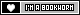## Udacity Programming Fundamental Lesson 5: Take a Break Mini-Project

### Jul 8, 2017 | Comments

Seeing that I am now unemployed and have a lot of time on my hands, I decided to utilize the free month of Udacity I received from Github’s Student Pack.

This mini-project’s goal is to create a simple program using Python to open a web browser at an interval of ten seconds three times in a row.

``````import time
import webbrowser

break_duration = 10
break_num = 3
break_count = 0

print("This program started at: " + time.ctime())

while(break_count < break_num):

time.sleep(break_duration)

webbrowser.open("http://edelgrace.me/blog")

break_count += 1``````

First I will explain the first two lines. In Python, there are many different modules that you can utilize to achieve specific things. Think of them as apps that you use on your phone. You have apps for calendars, contacts, games, social media, etc. Just like apps, we don’t really need to know how exactly modules works, just how to use them to get what we want. In this case, we use modules to get information about the time and to use the web browser on the computer.

``````import time
import webbrowser``````

Next, I initialize some variables. Think of this as giving something a name. For example, I am 22 years old as of writing this. This would be my age. In the code, I am declaring that the `break_duration` (how long to wait to open a web browser) is 10 seconds. The `break_num` determines how many breaks to take, which is 3. Lastly, the number of breaks taken so far, `break_count`, is 0.

``````break_duration = 10
break_num = 3
break_count = 0``````

This line will print out a message to the console with the `print` function. Inside print, it uses a function from the `time` module that we imported earlier. The function we use is `ctime()`, which grabs the value of the current time.

``print("This program started at: " + time.ctime())>``

The last chunk of code is a `while` loop. Think of while loops as a set of instructions to repeat until a condition is met. I will break this down line by line.

``````while(break_count < break_num):

time.sleep(break_duration)

webbrowser.open("http://edelgrace.me/blog")

break_count += 1``````

The first line determines the condition. This loop will keep running as long as the number of breaks taken (`break_count`) is less than the number of breaks that should be taken (`break_num`, which is 3). When this condition is not met, the code inside the while loop will stop running.

``while(break_count < break_num):``

Then we use the function `time.sleep()` to essentially wait for the number of seconds and essentially do nothing. From the official Python documentation, we can see that this function will accept numbers in terms of seconds, which is what we are using in `break_duration`.

``time.sleep(break_duration)``

Once the 10 seconds has elapsed, the next line of code will run. This uses the `webbrowser.open()` function. It essentially does what it says: open a web browser. Here, we indicate which site to open. In my case, we open up my blog for a quick break. :)

``webbrowser.open("http://edelgrace.me/blog")``

After that is all done, we have finished a break. Therefore, we increase the variable `break_num` by one to indicate that a break has been finished.

``break_count += 1``

The code then goes back to the start of the while loop and checks the condition. `break_count` is now 0+1=1 which is still less than `break_num` (which is 3). This repeats two more times until `break_count` is 3 and then the condition fails and the while loop is skipped over. Since there is no more code after the while loop, the program ends.

Category: Programming
Tags: